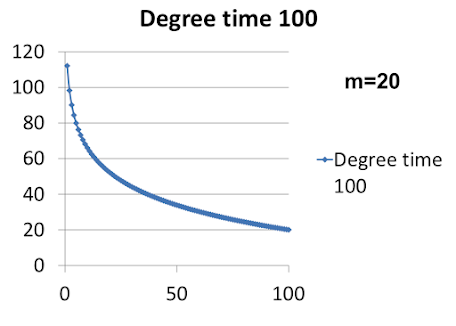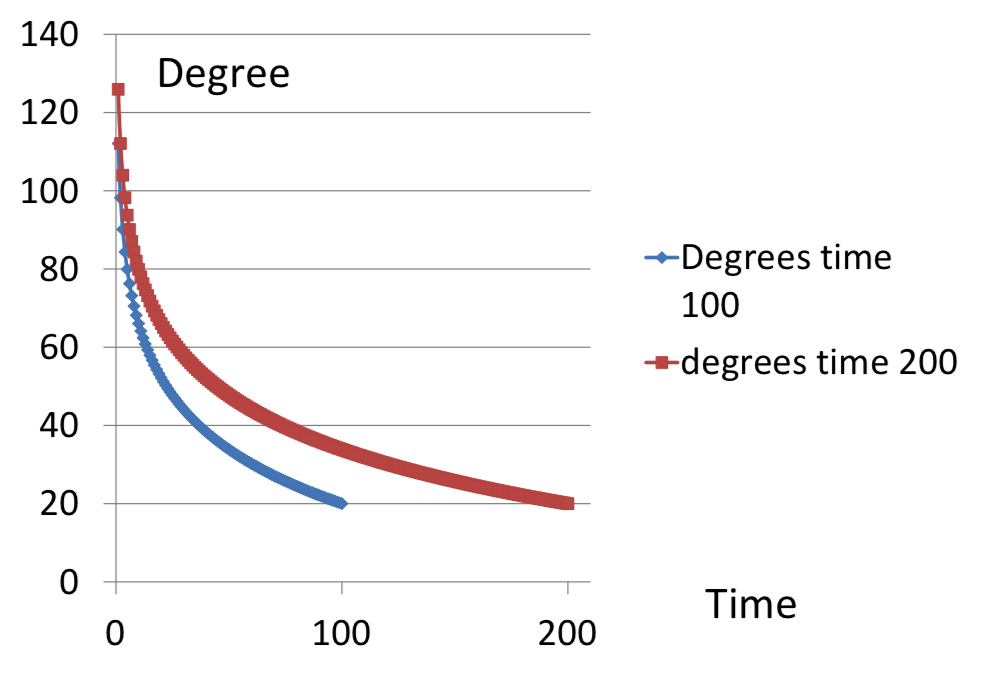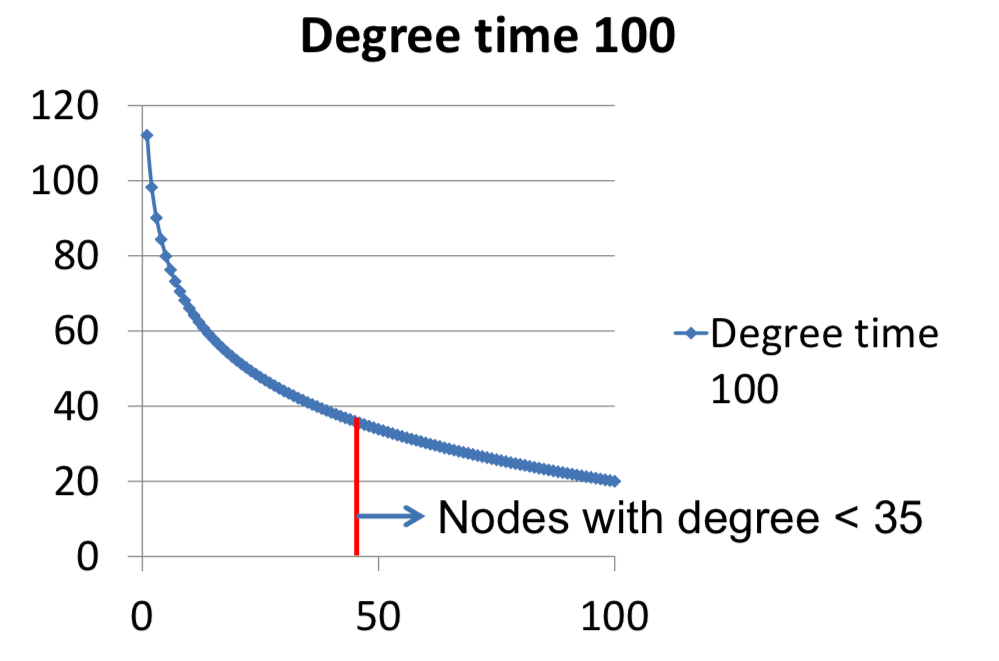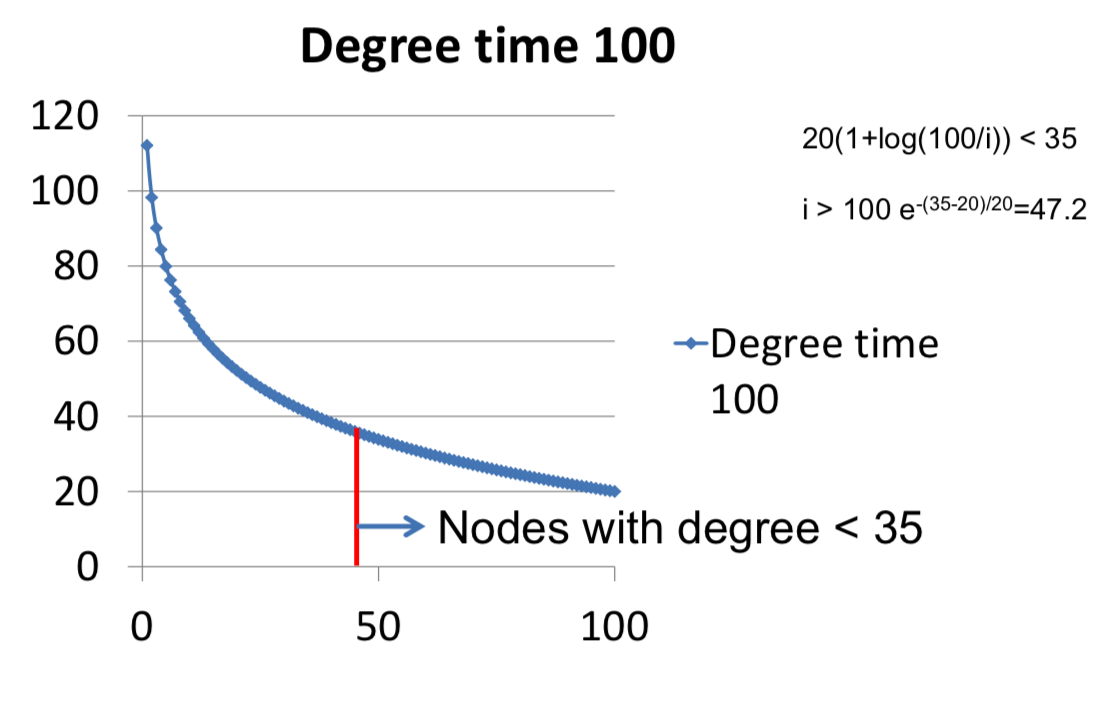# Matthew O. Jackson¶

## Santa Fe Institute, CIFAR¶

www.stanford.edu~jacksonm

# 3.1: Growing Random Networks¶

## What do they add?¶

### Realism(?)¶

Why do we care about having growing random networks?

One thing to emphasize just from the start is when we build models. We know that we're not going to try and capture everything in the world. And so realism is not usually a good reason for building a model to try and match reality. The reason that we build models, is to try and use as few moving parts as possible in order to reproduce the world. So the only reason we want to add this feature of growing random networks is not because that's the way the world is, but because this richer model might capture something in the real world that was not captured in the static models. So you shouldn't judge models based on realism, they're always wrong, the question is are they capturing reasonable elements and so here.

### Natural form of heterogeneity via age¶

The natural form of heterogeneity versus age is going to be useful in getting out more connected nodes and less connected nodes, and starting to see power laws and fat tails.

### A form of dynamics¶

So we're going to get a natural form of dynamics, and in particular, we're going to end up with a natural way of getting different degree distribution without just building them into the statistical distribution. So we could just assume that there's a distribution that has the features of reality.

### Natural way of varying degree distributions - not pre-specified as in static models¶

We want to do is see if build a model that will end up generating features that look like the real world, and this sort of dynamics is going to help quite a bit.

## Growing and Uniformly Random¶

What we're going to do is start by taking Erdos-Renyi random network situation, this simple benchmark will give us an idea of some of the techniques, then we can enrich is to have different kinds of formation processes besides the uniform at random.

• Each date a new node is born
• Forms m links to existing nodes
• Each node is chosen with equal likelihood

## Degree Distribution¶

• Start with m nodes fully connected
• New node forms m links to existing nodes
• An existing node has a probability m/t of getting new link each period
• m: the number of links being formed
• t: the existing number of nodes that are already there

## Distribution of Expected Degrees¶

Let's think about some node i that was born after the original m nodes that we started with that fully connected, and before some time t, now let's ask how many links has it, does it expect to have collected by time t?

Expected degree for node i born at $m<i<t$ is:

$m + m/(i+1) + m/(i+2) + ... + m/t$

$approx = m(1+log(t/i))$ (Harmonic numbers)

the first thing is that it forms some "m" links when it was born. Then in terms of expectations, the next day after it was born, if it was born at time "i", then the date now is "i+1", and there's some new node which is born, and so it has a chance "m/(i+1)" of getting these new links. Then at time, the next state there's "i+2", it's going to a number of new links are formed, and so forth.Nodes that have expected degree less than "d" at some time "t" are those such that $m(1+log(t/i)) < d$

so it is those $i > te^{-(d-m)/m}$

The fraction of nodes that have an expected degree of less than d, is given by this formula:

$F_t(d) = (t - te^{-(d-m)/m}) / t = 1 - e^{-(d-m)/m}$

## Degree distribution of growing random network¶

• Distribution of expected degrees is such that d-m is exponentially distributed (mean m)
• What about actual degrees?
• Good approximation for large t - need careful large numbers arguments